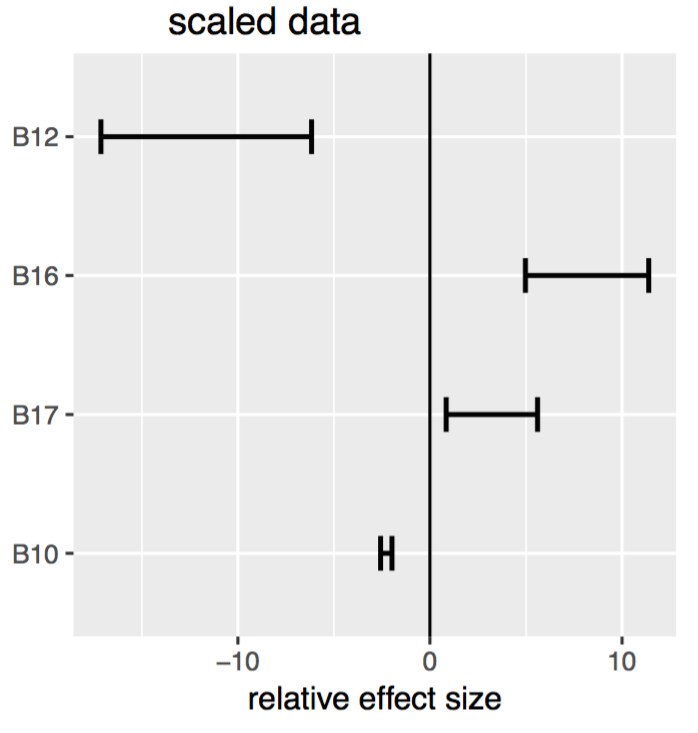One of the outputs of a Species Distribution Modelling experiment is the Variable Importance Plots (VIP), which can assist in understanding the contribution of environmental predictor variables to the model outputs. Our colleagues Assoc Prof Sama Low-Choy and Dr John Xie at Griffith University have written a special R function (VIPplot) for us to incorporate into the BCCVL to generate these plots. The plots are based on algorithm-specific outputs, and are currently available for the following algorithms: CTA, GAM, Maxent, GLM, GBM, RF and ANN. Depending on what is appropriate for each algorithm, the variable importance plots differ in assessment method.

#### Relative effect size

This plot is only included for the GLM algorithm.

The effect size measures the variable importance in terms of goodness-of-fit, and is here defined as the absolute values of the standardised regression coefficients (also referred to as the beta weights). Standardised coefficients refer to how many standard deviations the response variable will change per a standard deviation increase in the predictor variable. This reflects the rank ordering of the predictor variables with respect to the role they play in accounting for variability on the response variable. A negative effect size indicates that an increase in the value of the predictor (e.g. an increased temperature) results in a lower probability of occurrence, whereas a positive effect size indicates that an increase in the predictor variable results in an increased probability of occurrence. The graph represents the confidence interval of the effect size per predictor variable. If the confidence interval crosses the zero line, then the association is considered non-significant. Thus, only predictor variables with a confidence interval below or above zero are considered to be of significant importance. The variable with either the highest or lowest effect size is the most important variable. The effect size plot below indicates that B12 is the most important predictor variable, and B17 the least important, but all predictor variables have a significant effect on the response variable. It is important to note that the effect size assessment relies on the condition that all of the predictor variables are independent of another. Correlation among predictor variables makes it difficult to determine how much variability in the response variable can be accounted for by any single predictor variable. This is why the VIP output also includes a correlation matrix (see below).#### Akaike Information Criteria (AIC)

This plot is included for the GLM and GAM algorithms.

The AIC method measures the prediction performance in terms of information loss if a predictor variable would be excluded from the fitted model. It uses the Kullback-Leibler (K-L) information, which is a unique overall measure of discrepancies between a fitted model and the true model which generates the observed data. AIC is derived by minimizing the relative K-L information.#### Biomod function 'variable importance'

For the machine learning algorithms ANN and GBM, the AIC approach is not applicable because there is no model log-likelihood information available. Therefore, we have included the variable importance function that is implemented in the biomod2 package. This function uses a machine-learning approach once the models are trained to randomize one of the variables in each permutation and calculate a correlation score between the standard prediction and the new prediction. This score is considered to give an estimation of the variable importance in the model. The higher the value, the more importance the predictor variable has on the model. A value of 0 assumes no influence of that predictor. Note that this method does not account for interactions between variables and should be considered more as an information tool for each model independently.#### Variable importance score for CTA algorithm

For tree-based models, the variable importance is measured by the total decrease in node impurities from splitting on the variable, averaged over all trees. For classification trees, this impurity is measured by the Gini index. The VIP output for the Classification Tree algorithm in the BCCVL includes the tree plot as well as the variable importance score plot.#### Correlation matrix

This matrix is currently only included for the GLM and Maxent algorithms.

This matrix shows the linear correlation among predictor variables included in the model. This can be used to ensure that the included variables in the model are not too highly correlated which might bias the model outputs. For each combination of predictor variables the correlation is indicated as being either strongly negative (dark green), weakly negative (light green), weakly positive (light pink) or strongly positive (dark pink). The diagonal of the matrix represents the relationship of each variable with itself which is obviously always 1.